Next: The Hamilton-Jacobi Equation for Up: Laser-driven particle mechanics Previous: Advantage of the Hamiltonian   Contents

# The Dynamical Phase

The most important scalar function for a dynamical system is its dynamical phase. This phase is the (value of) the integral, Eq.(1), evaluated for a worldline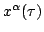which starts at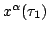, which satisfies the Lagrange equations of motion, and which therefore extremizes this integral. Let us designate this extremal value by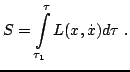This integral is a function of the worldline's termination point which we take to be. If there is only one worldlines betweenand, as is usually the case, theis a single valued function of the termination point. This is depicted in Figure 3. (If there were several such worldlines, thenwould be multivalued.) Thusis a function of the location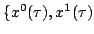,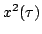,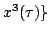of that termination point. It also is a function of the parameter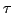which one uses to parametrize the worldline. Thus one has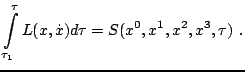Next: The Hamilton-Jacobi Equation for Up: Laser-driven particle mechanics Previous: Advantage of the Hamiltonian   Contents
Ulrich Gerlach 2005-11-07